﻿ 舰炮驻退机流场研究
 舰船科学技术2019, Vol. 41Issue (8): 149-153PDF

Flow field research on recoil brake of a naval gun
LIU Da-qing, LI Xiang, QIU Qun-xian
The 713 Research Institute of CSIC, Zhengzhou 450015, China
Abstract: Recoil brake is an important part of a naval gun recoil mechanism, The traditional design of recoil brake has large errors and could get very limited data. The whole flow field can not be obtained. The problem of long recovery time for a naval gun will appear in long-term launch shock test. With regard to this problem, the calculation of traditional design method is inconsistent with the result of firing test. In this paper, the CFD method is used to re-analysis the flow field of the recoil brake. Through computer simulation and analysis, the variation law of internal flow field in recoil brake is studied, and the practical engineering problem are solved by using the hydrodynamics theory. It also provides a reference for solving this problem of long recovery time.
Key words: recoil brake     dynamic mesh     multiphase modeling     hydrodynamics
0 引　言

1 驻退机模型建立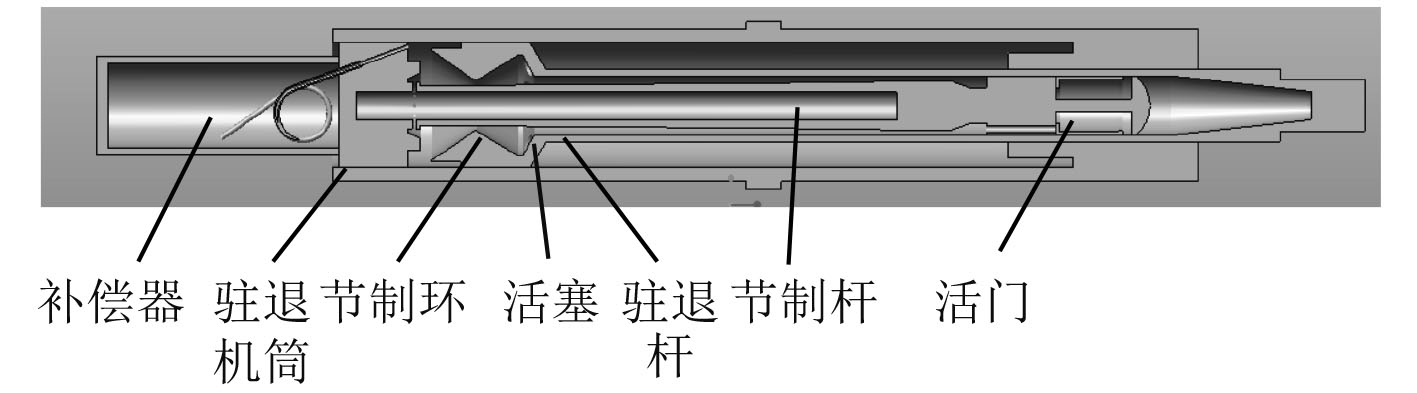图 1 驻退机结构模型 Fig. 1 Structural diagram of recoil mechanism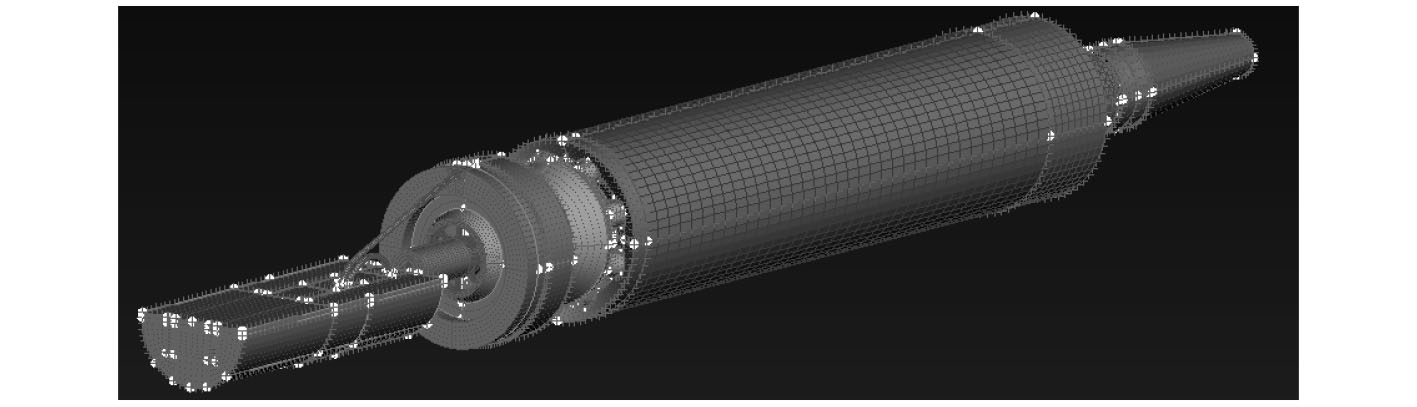图 2 驻退机网格图 Fig. 2 Meshing diagram of recoil mechanism
2 驻退机内部流场运动规律

 $\begin{split} & \rho \left\{ {\frac{{\partial \varPhi }}{{\partial t}} + \frac{{\partial \mu \varPhi }}{{\partial x}} + \frac{{\partial \nu \varPhi }}{{\partial y}} + \frac{{\partial \omega \varPhi }}{{\partial z}}} \right\} = \frac{\partial }{{\partial x}}\left\{ {\Gamma \frac{{\partial \varPhi }}{{\partial x}}} \right\}+ \\ & \frac{\partial }{{\partial y}}\left\{ {\Gamma \frac{{\partial \varPhi }}{{\partial y}}} \right\} + \frac{\partial }{{\partial z}}\left\{ {\Gamma \frac{{\partial \varPhi }}{{\partial z}}} \right\} + S \text{。} \end{split}$

 $\rho \frac{{{\rm{D}}k}}{{{\rm{D}}t}} = \frac{\partial }{{\partial {x_i}}}\left[ {\left( {\mu + \frac{{{\mu _t}}}{{{\sigma _k}}}} \right)\frac{{\partial k}}{{\partial {x_i}}}} \right] + {G_k} + {G_b} - \rho \varepsilon - {Y_M}{\text{，}}$
 $\rho \frac{{{\rm{D}}\varepsilon }}{{{\rm{D}}t}} = \frac{\partial }{{\partial {x_i}}}\left[ {\left( {\mu + \frac{{{\mu _t}}}{{{\sigma _k}}}} \right)\frac{{\partial \varepsilon }}{{\partial {x_i}}}} \right] + {C_{1\varepsilon }}\frac{\varepsilon }{k}\left( {{G_k} + {C_{3\varepsilon }}{G_b}} \right) - {C_{2\varepsilon }}\rho \frac{{{\varepsilon ^2}}}{k}{\text{。}}$

3 驻退机流场数值模拟分析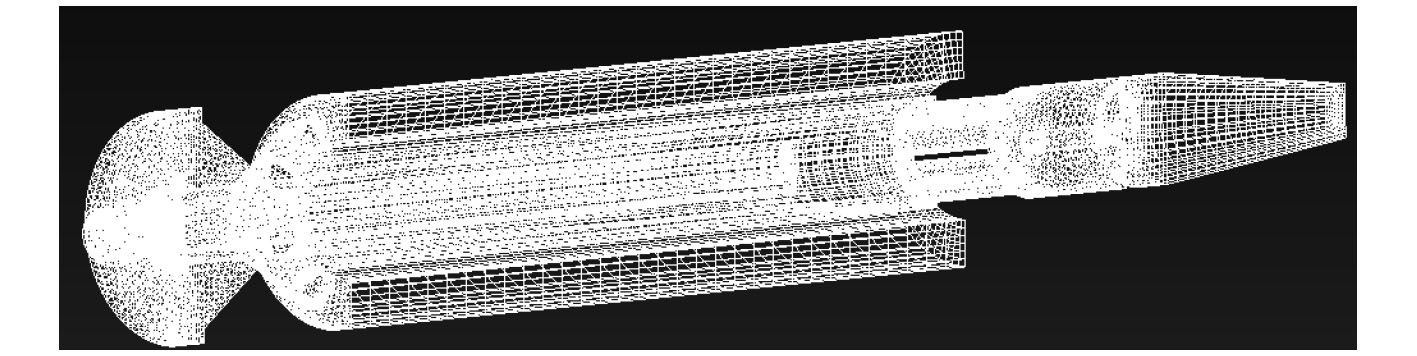图 3 不带补偿器的驻退机网格模型 Fig. 3 Meshing diagram of recoil mechanism without compensator
3.1 不带补偿器的驻退机单发后坐复进模拟分析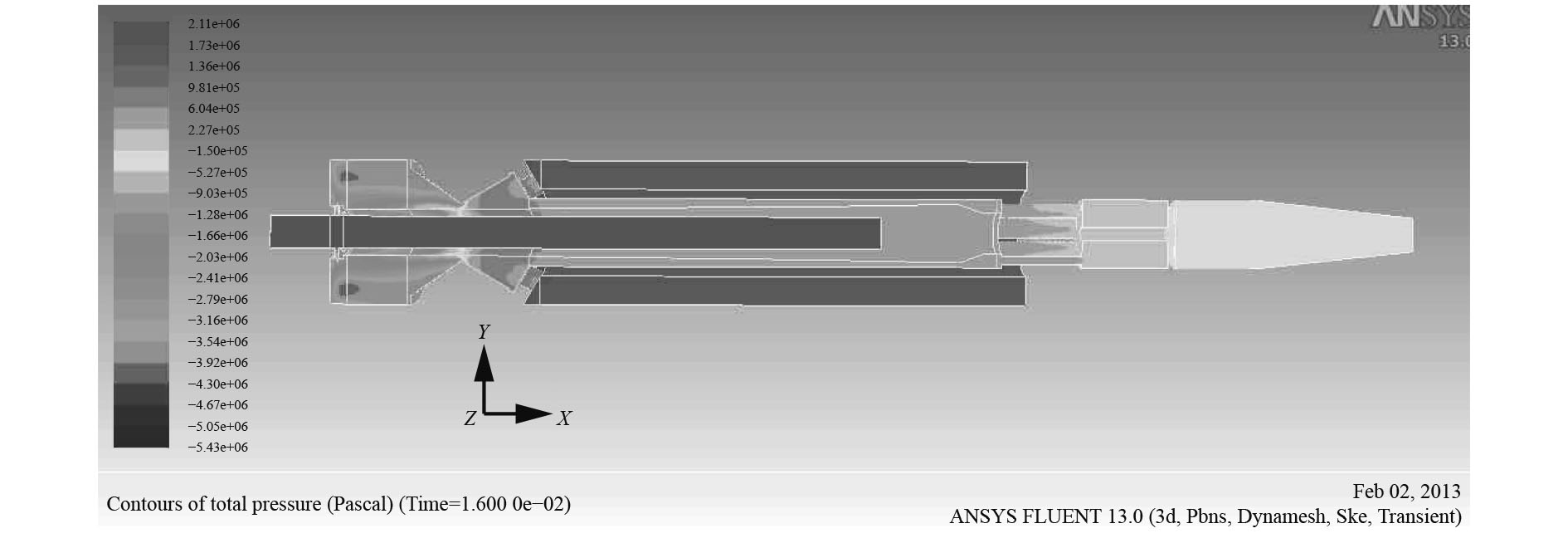图 4 0.016 s时压力云图 Fig. 4 Contours of dynamic pressure at 0.016 s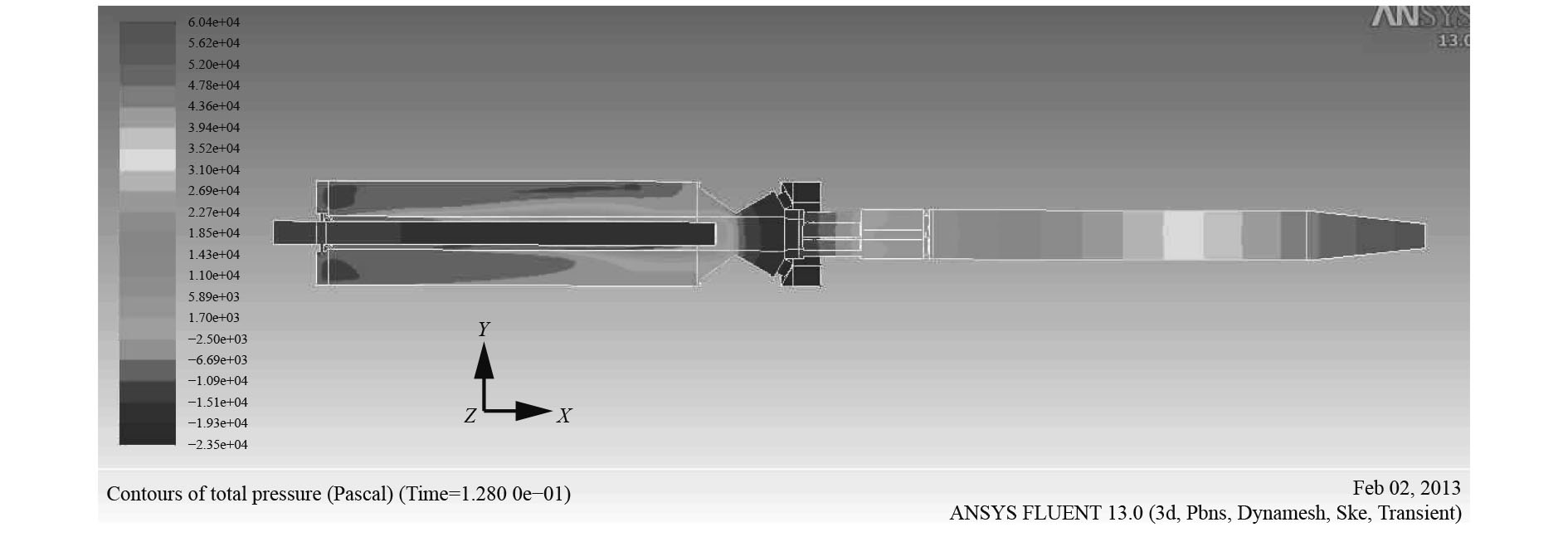图 5 0.128 s时压力云图 Fig. 5 Contours of dynamic pressure at 0.128 s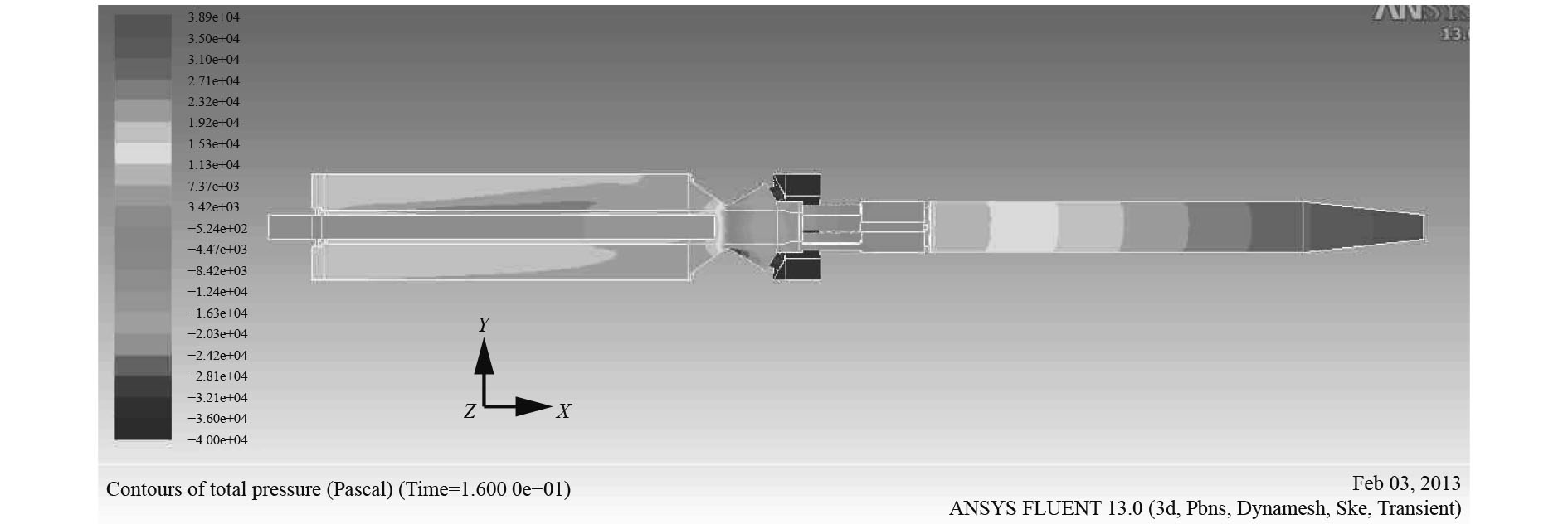图 6 0.16 s时压力云图 Fig. 6 Contours of dynamic pressure at 0.16 s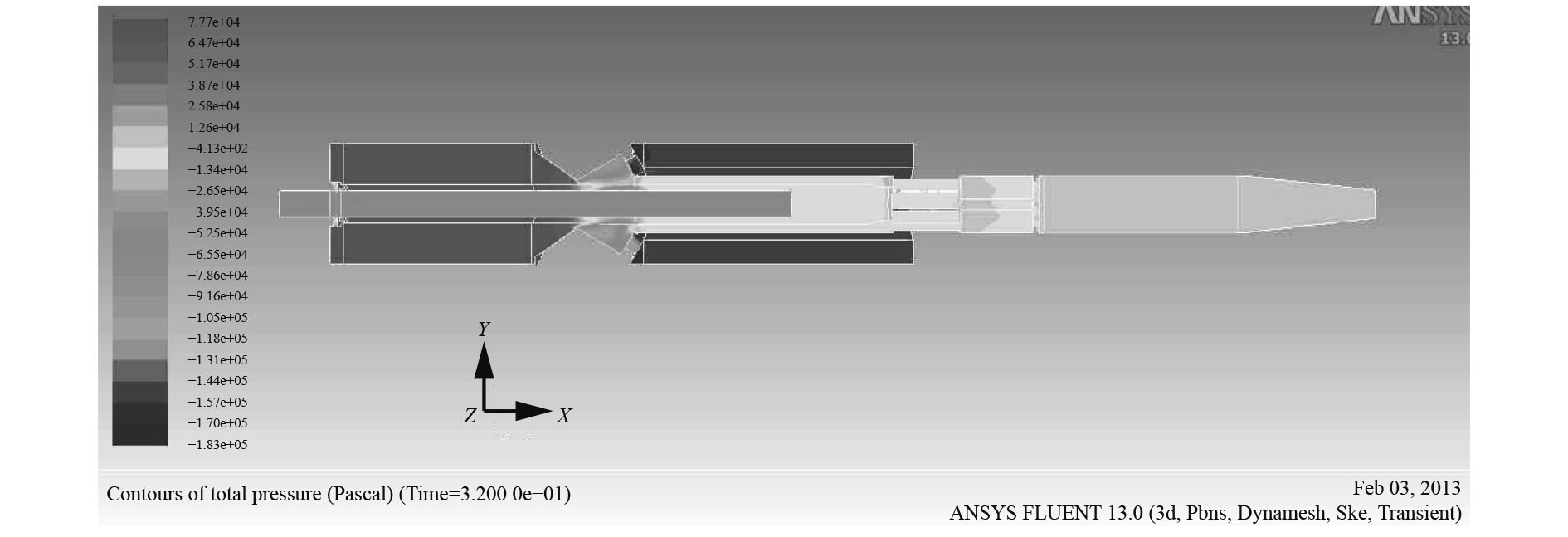图 7 0.32 s时压力云图 Fig. 7 Contours of dynamic pressure at 0.32 s

3.2 带补偿器的驻退机单发后坐复进模拟分析

1）驻退机内部流场体积分数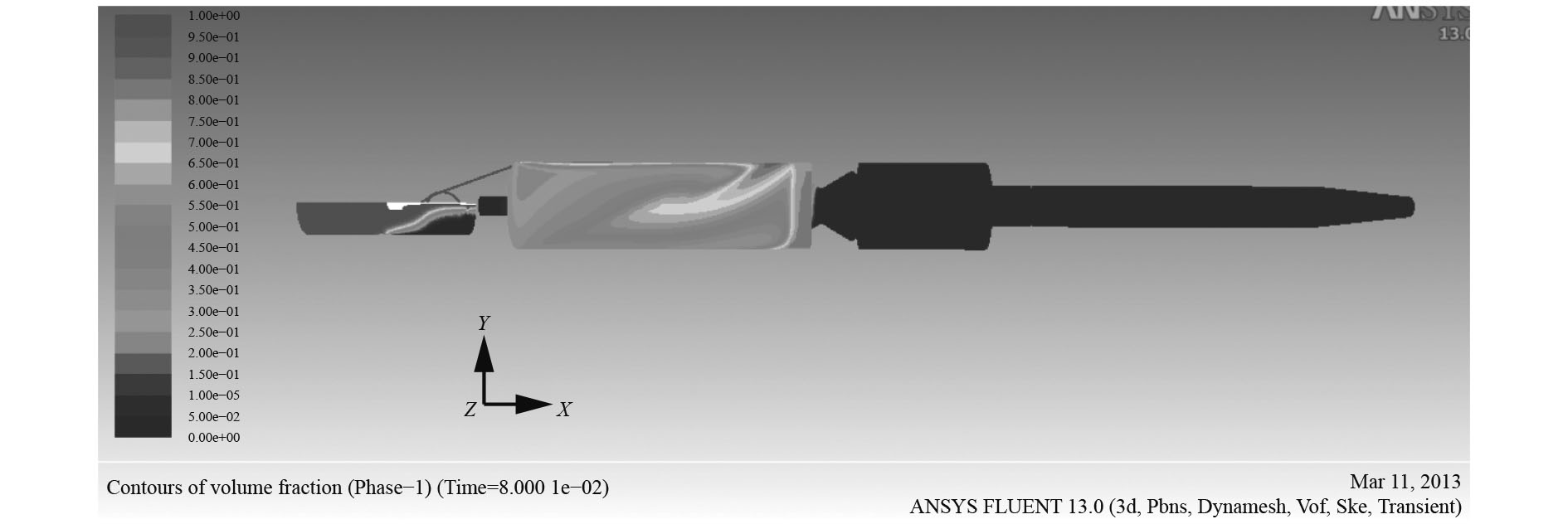图 8 0.08 s时体积分数云图 Fig. 8 Contours of volume fraction at 0.08 s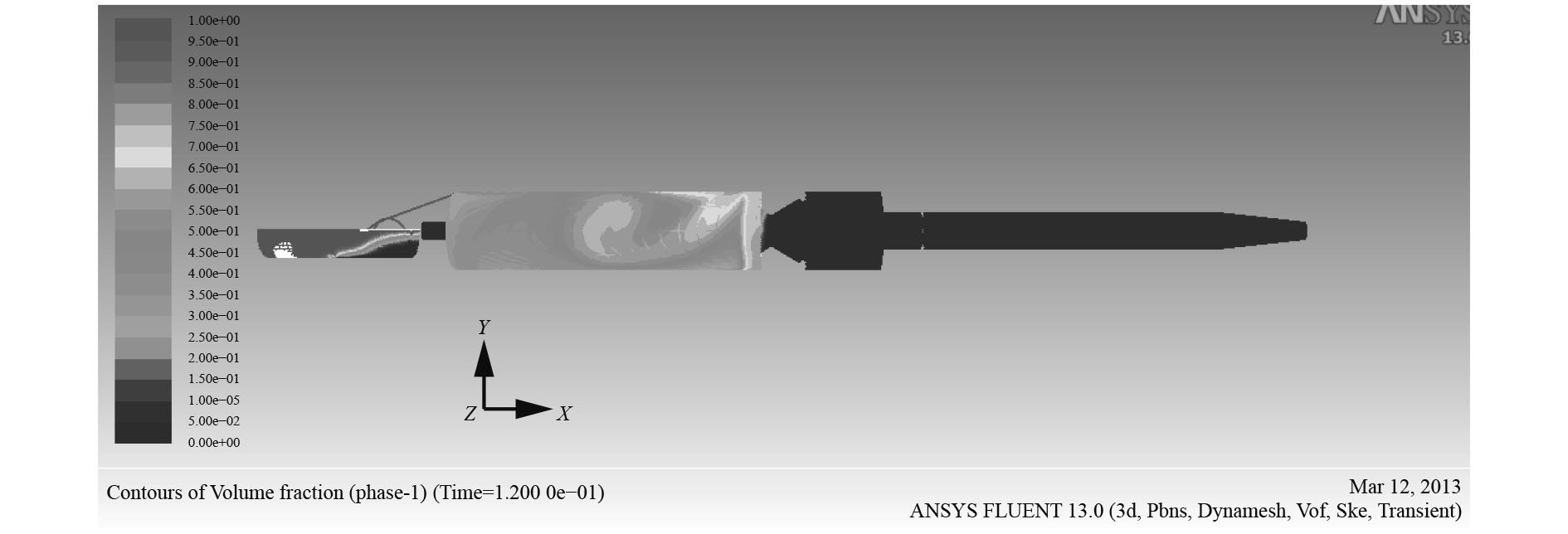图 9 0.12 s时体积分数云图 Fig. 9 Contours of volume fraction at 0.12 s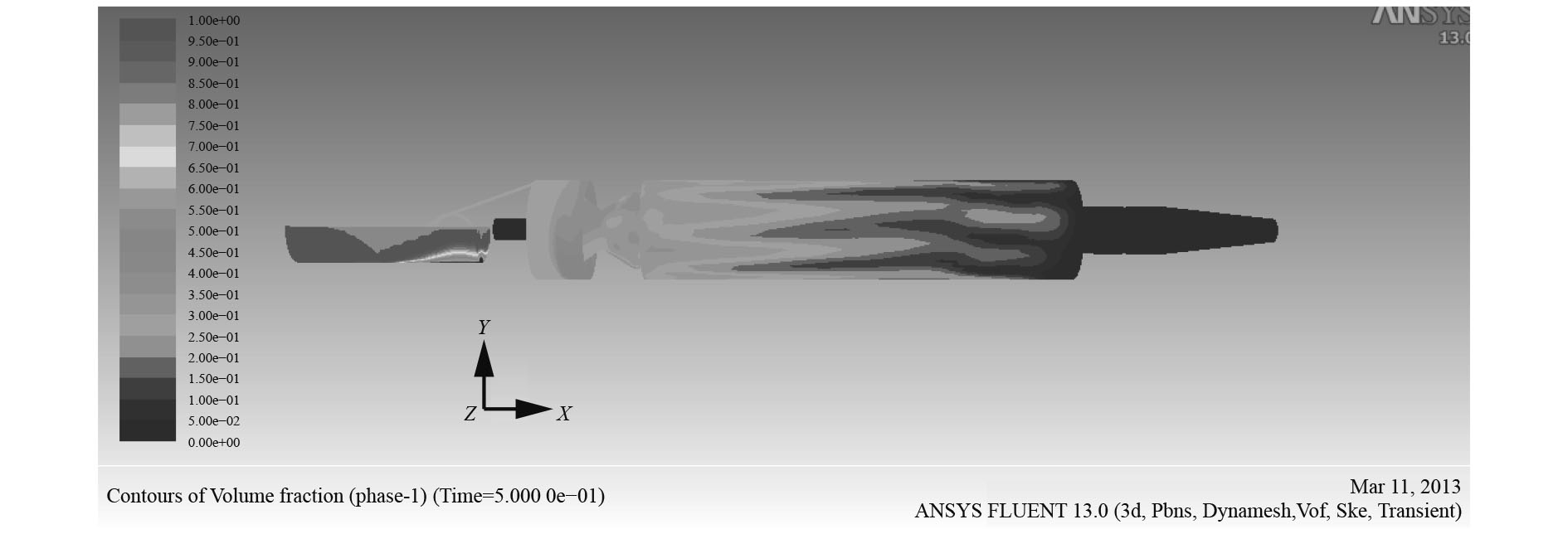图 10 0.5 s时体积分数云图 Fig. 10 Contours of volume fraction at 0.5 s

2）驻退机内部流场压力分析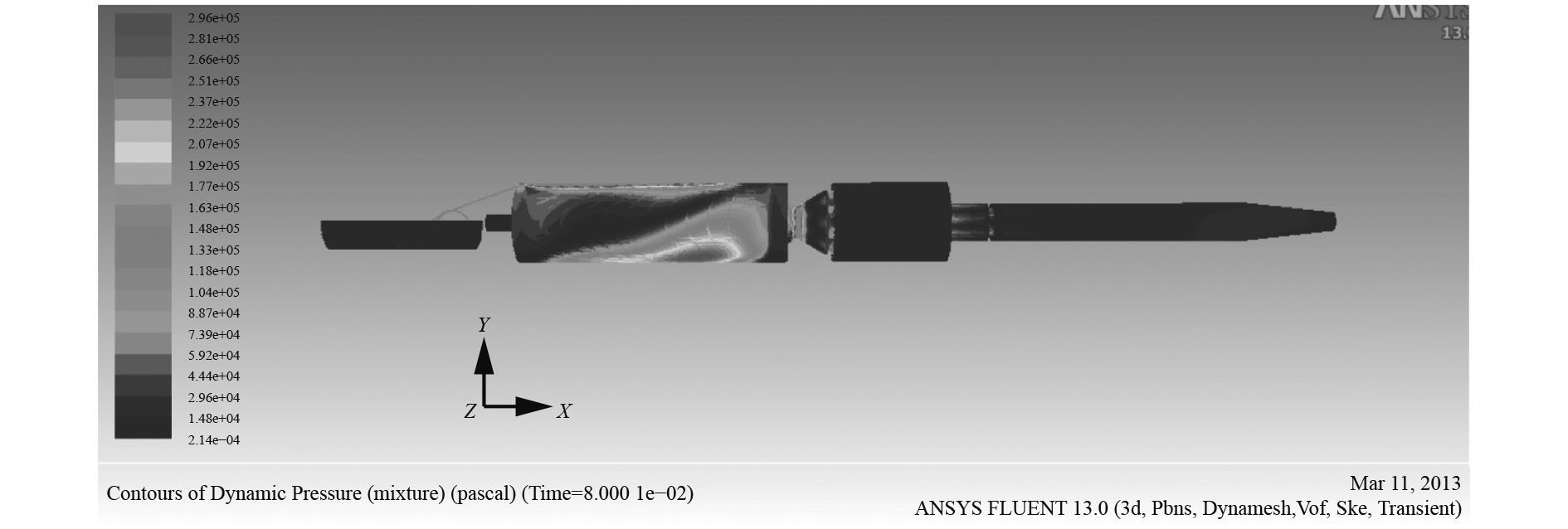图 11 0.08 s时压力云图 Fig. 11 Contours of dynamic pressure at 0.08 s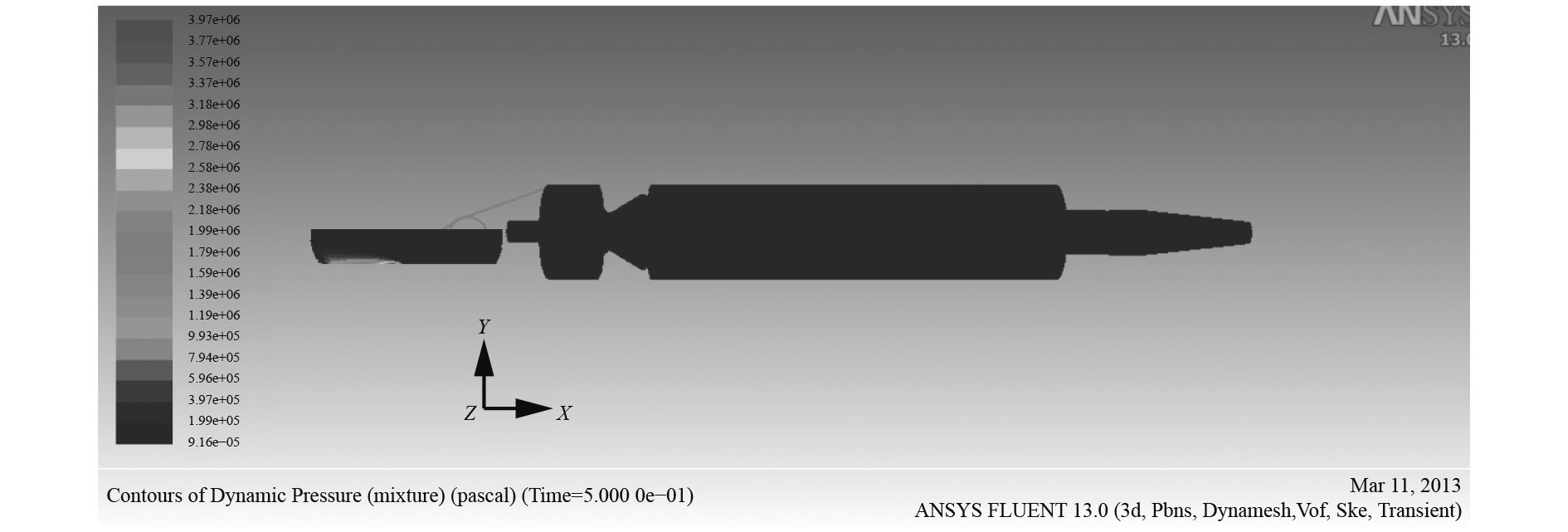图 12 0.5 s时压力云图 Fig. 12 Contours of dynamic pressure at 0.5 s

3.3 带补偿器的驻退机连发后坐复进模拟分析

1）驻退机内部流场体积分数和有效粘度分析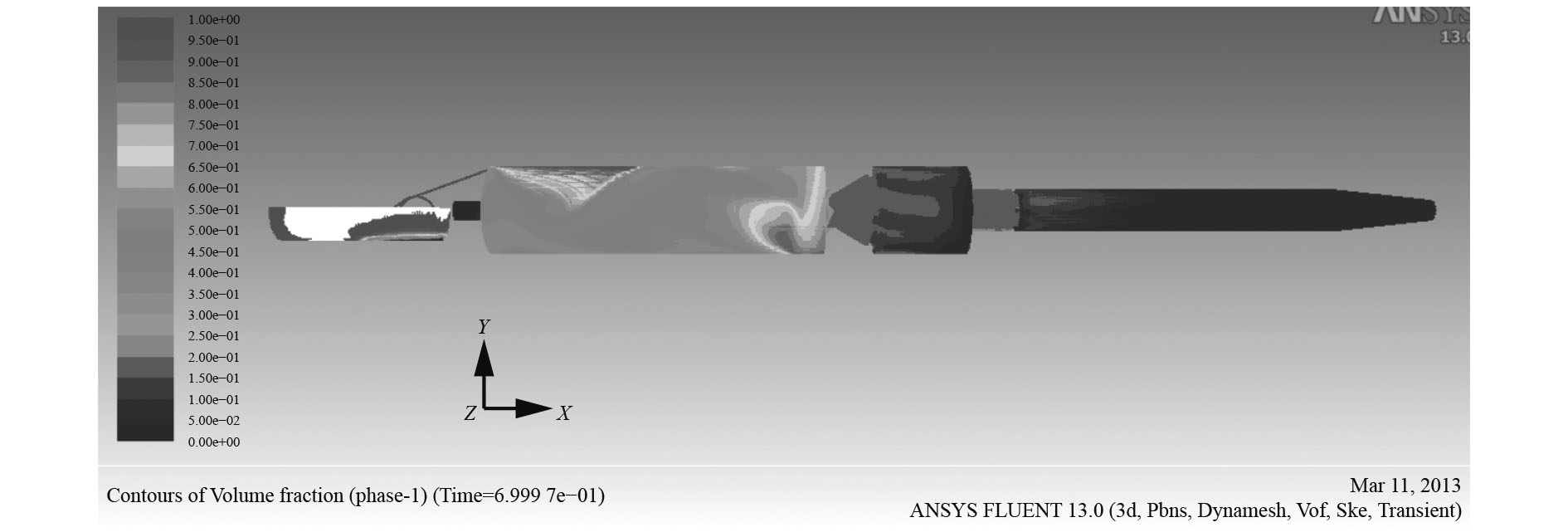图 13 0.7 s时体积分数云图 Fig. 13 Contours of volume fraction at 0.7 s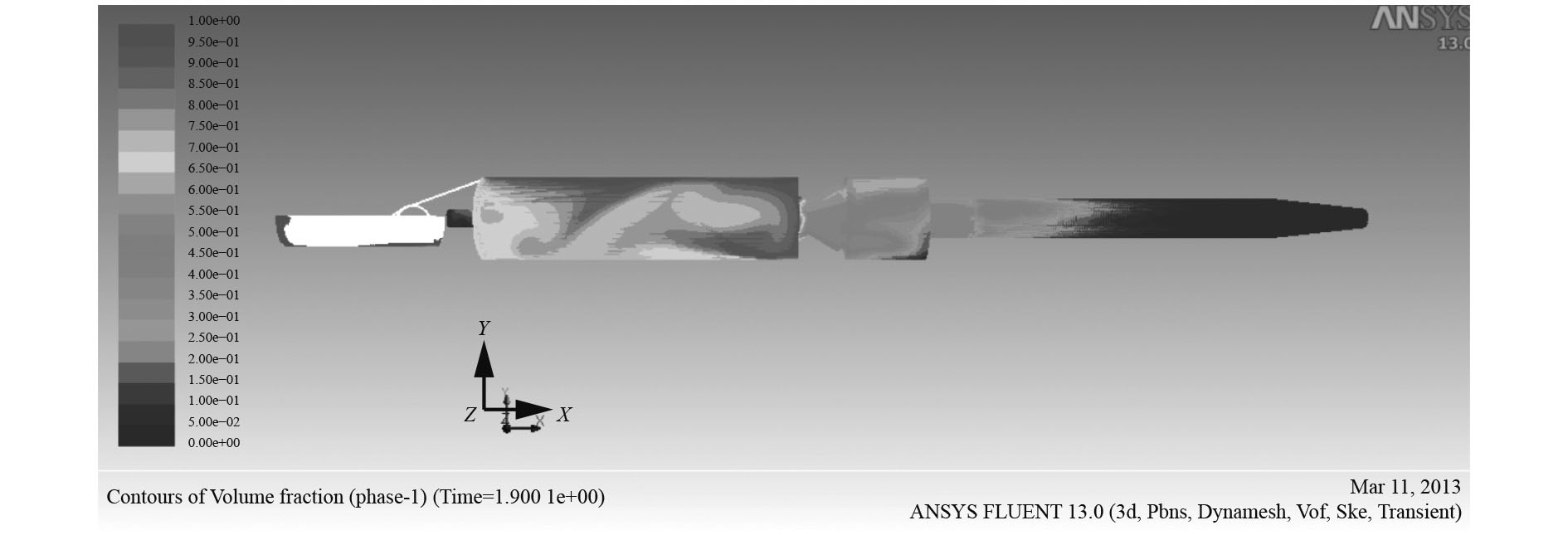图 14 1.9 s时体积分数云图 Fig. 14 Contours of volume fraction at 1.9 s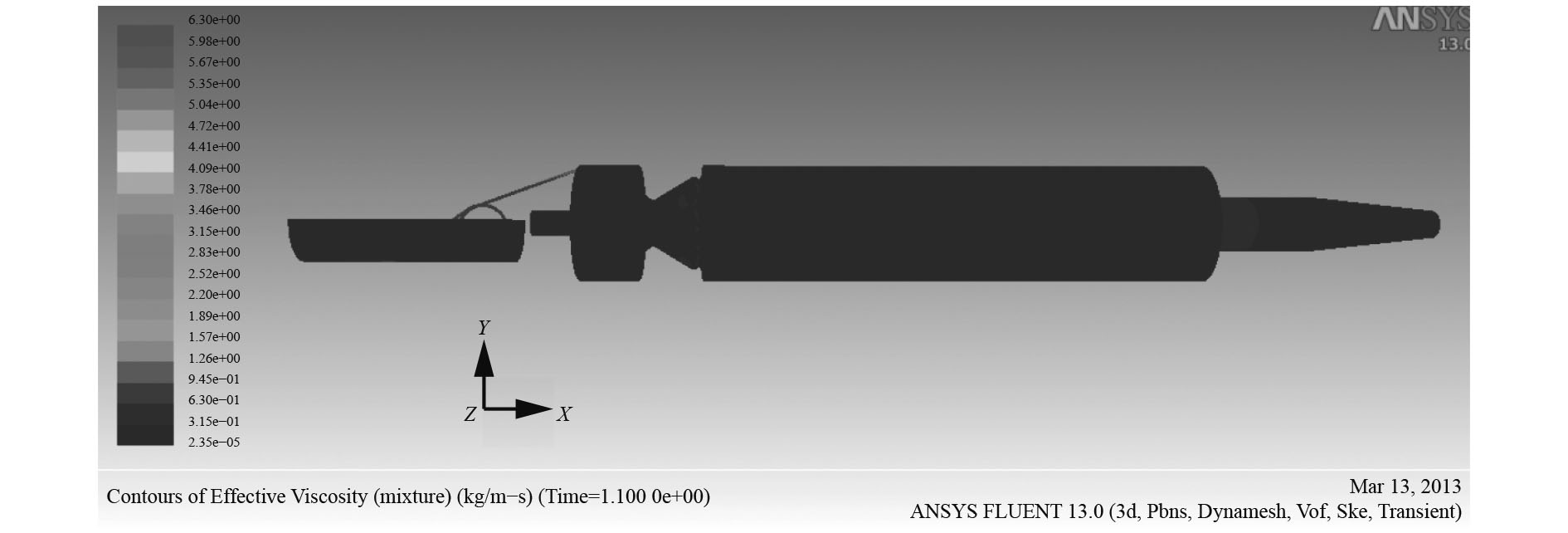图 15 1.1 s时有效粘度云图 Fig. 15 Contours of effective viscosity at 1.1 s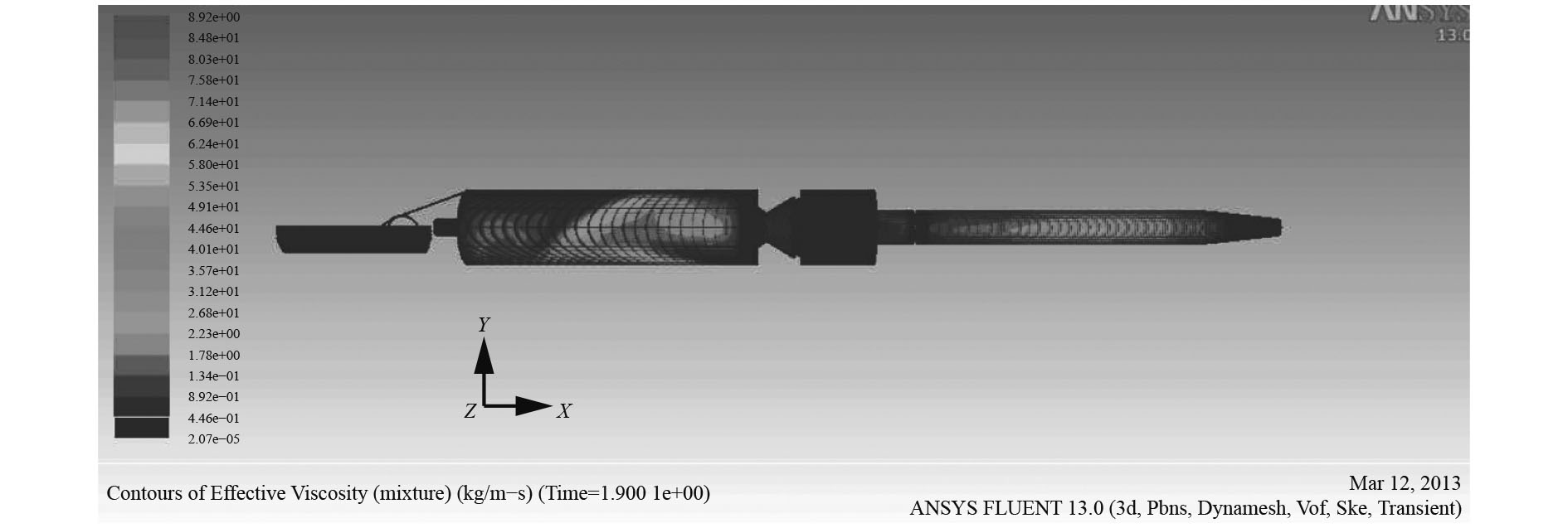图 16 1.9 s时有效粘度云图 Fig. 16 Contours of effective viscosity at 1.9 s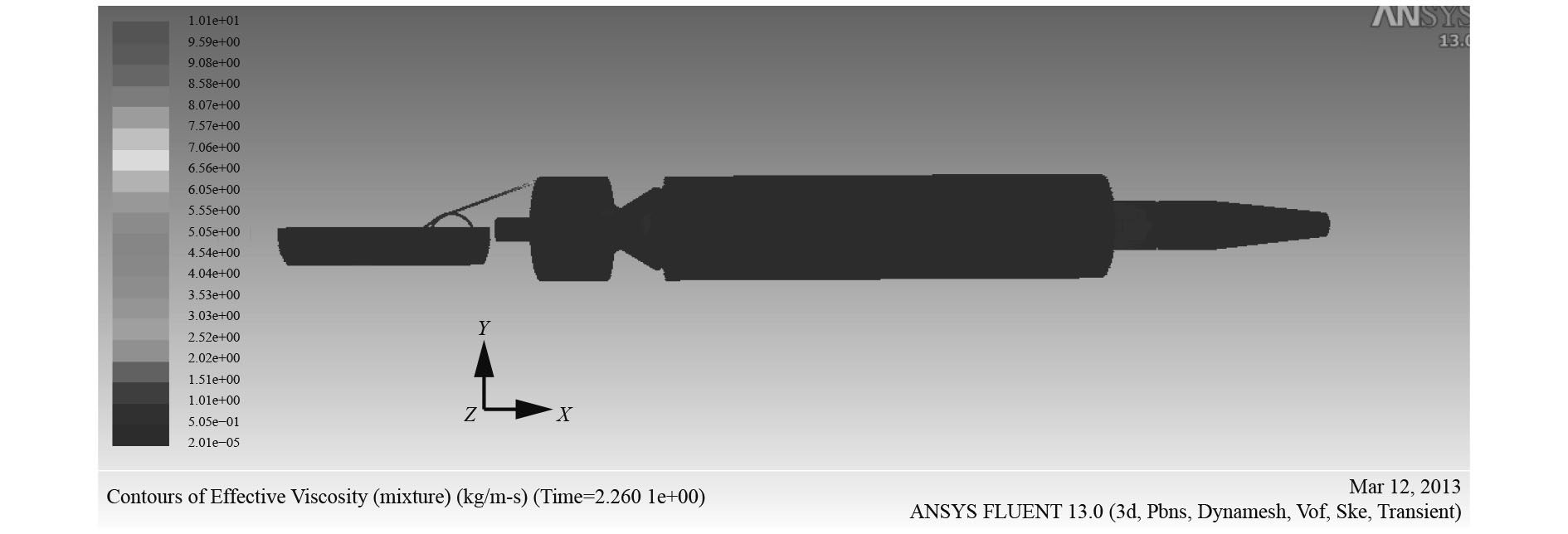图 17 2.26 s时有效粘度云图 Fig. 17 Contours of effective viscosity at 2.26 s

2）驻退机内部流场压力分析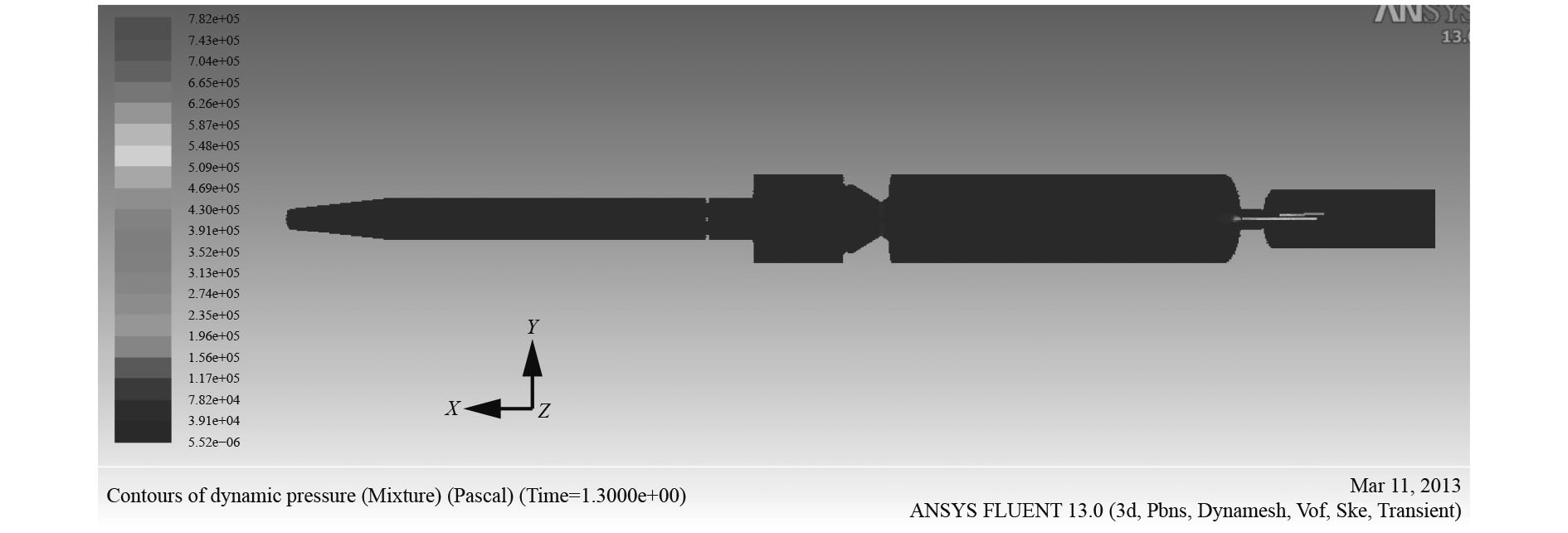图 18 1.3 s时压力云图（顶视图） Fig. 18 Contours of dynamic pressure at 1.3 s（top view）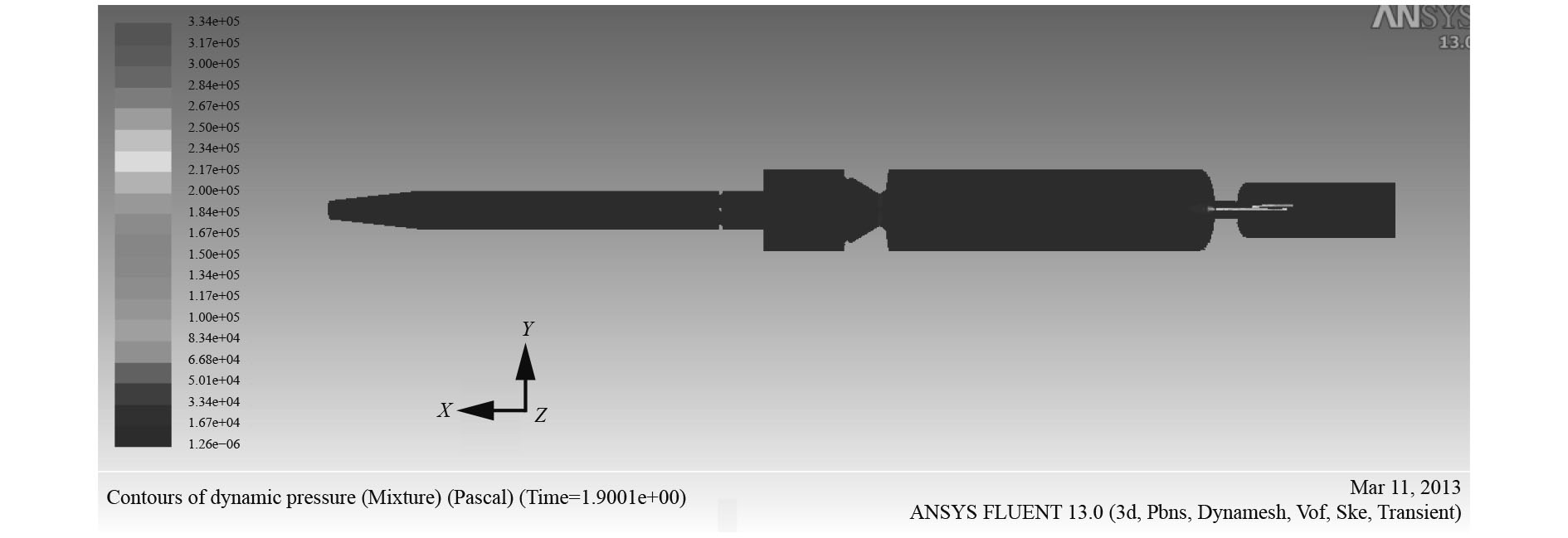图 19 1.9 s时压力云图（顶视图） Fig. 19 Contours of dynamic pressure at 1.9 s（top view）
4 结　语

  高跃飞. 火炮反后坐装置设计[M]. 北京: 国防工业出版社, 2010.  胡胜海, 宋洪侠, 曲东越, 等. 舰炮缓冲技术的研究[J]. 哈尔滨工程大学学报, 2000, 21(1): 76-78. HU Sheng-hai, SONG Hong-xia, QU Dong-yue, et al. Research on Buffering Technique of Nun Gun[J]. Journal of Harbin Engineering University, 2000, 21(1): 76-78. DOI:10.3969/j.issn.1006-7043.2000.01.018  郑建国. 火炮制退机性能的数值预测[J]. 火炮发射与控制学报, 2000(2): 10-13. ZHENG Jian-guo. Digital value prediction for perfomance of gun muzzle brake[J]. Journal of gun launch & Control, 2000(2): 10-13. DOI:10.3969/j.issn.1673-6524.2000.02.003  杨猛. 计算流体力学两相流流动的模拟及两相流模型的研究[D]. 天津: 天津大学. 2005: 36-38. YANG Meng. Two-phase flow CFD simulations and its study[D]. Tianjin: Tianjin University 2005: 36-38.  孙磊. 含动边界的燃气射流数值模拟[D]. 南京: 南京理工大学. 2006: 8-11. SUN Lei. Numercal simulation of gas plume including movable bullet[D]. Nanjing: Nanjing university of technology. 2006: 8-11.  翟晓超, 陈亚军, 姜毅. 动网格在仿真发射装置内弹道中的应用[J]. 现代防御技术, 2006, 34(2): 24-28. ZHAI Xiao-chao, CHEN Ya-jun, JIANG Yi. Using dynamic grid to simulate interior trajectory of the launching container[J]. Modern defence technology, 2006, 34(2): 24-28. DOI:10.3969/j.issn.1009-086X.2006.02.006  周成. 某火炮驻退机流场数值模拟及冲蚀磨损预测[D]. 南京: 南京理工大学. 2006: 21-29. ZHOU Cheng. Numerical simulation of flow field and prediction of erosion wear in a gun recoil mechanism[D]. Nanjing: Nanjing university of technology. 2006: 21-29.  狄长春, 顾赵强, 崔凯波, 等. 基于液固两相流的某驻退机冲蚀磨损过程模拟研究[J]. 润滑与密封, 2017, 42(2): 81-86. DI Chang-chun, GU Zhao-qiang, CUI Kai-go, et al. Simulation Research on Erosion Wear of mechanism based on liquid-solid Two-phase flow[J]. Lubrication engineering, 2017, 42(2): 81-86. DOI:10.3969/j.issn.0254-0150.2017.02.016  陈朝军. 火炮驻退机流场数值模拟[D]. 南京: 南京理工大学. 2013: 47-50. CHEN Chao-jun. Numerical simulation of flow field in a gun recoil mechanism[D]. Nanjing: Nanjing university of technology. 2013: 47-50.